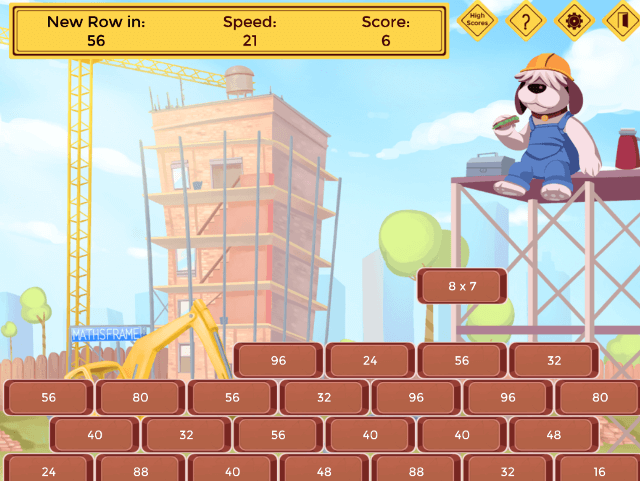## Monty's Maths Wall - Tablet Version

Use the arrow keys to guide your brick and destroy the wall or if you are using a tablet, tap either side to direct your brick and both sides to make it fall faster.

A great game for practising a wide range of mathematical skills. Levels are based on objectives from the new maths curriculum from Year 1 to Year 6. Topics include: multiplication, addition, reading numbers, subtraction, fractions of numbers, Roman numerals, division, converting fractions to decimals and percentages and simplifying fractions.

You can choose to play a single level, a selection of levels, or choose all the objectives from a year group (within the same topic). There is a full list of levels below.### Game Objectives

Multiplication

M.1 Recall and use doubles to all number to 10 (Y1)

M.2 2 times table (Y2)

M.3 5 times table (Y2)

M.4 10 times table (Y2)

M.5 Derive and use doubles of simple two-digit numbers (Y2)

M.6 3 times table (Y3)

M.7 4 times table (Y3)

M.8 8 times table (Y3)

M.9 Double numbers up to 100 (Y3)

M.10 Doubles of multiples of 50 up to 500 (Y3)

M.11 6 times table (Y4)

M.12 7 times table (Y4)

M.13 9 times table (Y4)

M.14 11 times table (Y4)

M.15 12 times table (Y4)

M.16 Double 3 digit numbers (Y4)

M.17 Double decimals (1 decimal place) (Y4)

M.18 Recognise square numbers (up to 15 squared) (Y5)

M.19  Recognise cube numbers (up to 10 cubed) (Y5)

M.20 Double decimals (2 decimal places) (Y5)

A.1 Add numbers up to 10 (Y1)

A.2 Add numbers up to 20 (Y1)

A.3 Add two-digit numbers and ones (Y2)

A.4 Add two-digit numbers and tens (Y2)

A.6 Add three one-digit numbers (Y2)

A.7 Add three-digit numbers and ones (Y3)

A.8 Add three-digit numbers and tens (Y3)

A.9 Add three-digit numbers and hundreds (Y3)

A.10 Add combinations of two and three-digit numbers (Y4)

A.11 Add combinations of decimals (1dp) (Y4)

A.12 Add combinations of three-digit numbers (Y5&6)

A.13 Add combinations of decimals (2dp) (Y5&6)

R.N.1 Read numbers to 20 in numerals and words (Y1)

R.N.2  Read numbers to 100 in numerals and words (Y2)

Subtraction

S.1 Subtract numbers from up to 10 (Y1)

S.2 Subtract numbers from up to 20 (Y1)

S.3 Subtract ones from a two-digit number (Y2)

S.4 Subtract tens from a two-digit number (Y2)

S.5 Subtract a two digit number from a two-digit number

S.6 Subtract from 100 (multiples of 10) (Y3)

S.7 Subtract from 100 (multiples of 5) (Y3)

S.8 Subtract from 100 (Y3)

S.9 Subtract from 1000 (multiples of 5)

S.10 Subtract ones from three-digit numbers (Y3)

S.11 Subtract tens from three-digit numbers (Y3)

S.12 Subtract hundreds from three-digit numbers (Y3)

S.13 Subtract decimals (1dp) from 1 (Y4&5)

S.14 Subtract decimals (1dp) from 10 (Y4&5)

S.15 Subtract two-digit numbers from three-digit numbers (Y4)

S.16 Subtract combinations of decimals (Y4)

S.17 Subtract a decimal (2dp) from 1 (Y5&6)

S.18 Subtract combination of three-digit numbers (Y5&6)

S.19 Subtract combinations of decimals (2dp) (Y5&6)

Fractions of Numbers

FN.1 1/2 of numbers up to 20 (whole answers)

FN.2 1/2 of numbers up to 40 (whole answers)

FN.3 1/2 of numbers up to 100 (whole answers)

FN.4 1/4 of numbers up to 100 (whole answers)

FN.5 Unit fractions of up to 50 (whole answers)

FN.6 1/10 of multiples of 10 (e.g. 1/10 of 80)

FN.7 Multiples of 1/10 of multiples of 10 (e.g. 6/10 of 40)

FN.8 Simple multi-part fractions of up to 100 (whole answers)

FN.9 Harder multi-part fractions of up to 100 (whole answers)

FN.10 1/10 of numbers up to 100 (inc. decimal answers)

FN.11 Multiples of 1/10 of numbers up to 100 (inc. decimal answers)

FN.12 Unit fraction of up to 20 (inc. decimal answers)

Roman Numerals

RomN.1 Read Roman numerals to 12

RomN.2 Read Roman numerals to 50

RomN.3 Read Roman numerals to 100

RomN.4 Read Roman numerals to 1000

RomN.5 Recognise years written in Roman numerals

Division

D.1 Half of numbers to 20 (Y1)

D.2 Division facts for the 2 times table (Y2)

D.3 Division facts for the 5 times table (Y2)

D.4 Division facts for the 10 times table (Y2)

D.5 Deriving halves of two-digit even numbers (Y2)

D.6 Division facts for the 3 times table (Y3)

D.7 Division facts for the 4 times table (Y3)

D.8 Division facts for the 8 times table (Y3)

D.9 Halve numbers up to 200 (Y3)

D.10 Division facts for the 6 times table (Y4)

D.11 Division facts for the 7 times table (Y4)

D.12 Division facts for the 9 times table (Y4)

D.13 Division facts for the 11 times table (Y4)

D.14 Division facts for the 12 times table (Y4)

D.15 Halve decimals (1dp)

D.16 Halve decimals (2dp)

Convert Fractions to Decimals

CFD.1 Unit Fractions (1/2, 1/4, 1/5, 1/10, 1/100)

CFD.2 Multi-part Fractions (e.g. 3/4, 2/5, 8/10, 23/100)

CFD.3 Harder unit fractions (as per level 1 plus 1/3, 1/6 1/8)

CFD.4 Harder multi-part fractions (e.g. 2/3, 5/6, 3/8)

CFD.5 Improper fractions

Convert Fractions to Percentages

CFP.1 Unit Fractions (1/2, 1/4, 1/5, 1/10, 1/100)

CFP.2 Multi-part Fractions (e.g. 3/4, 2/5, 8/10, 23/100)

CFP.3 Harder unit fractions (as per level 1 plus 1/3, 1/6 1/8)

CFP.4 Harder multi-part fractions (e.g. 2/3, 5/6, 3/8)

CFP.5 Improper fractions

Simplify Fractions

SF.1 Find fractions in their simplest form (unit fraction answers)

SF.2 Find fractions in their simplest form (inc multi-part fraction answers)